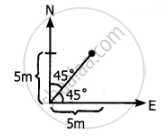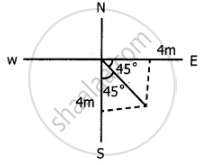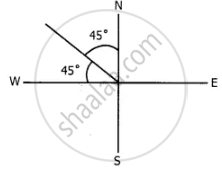Tamil Nadu Board of Secondary EducationHSC Science Class 11th

# Consider the x-axis as representing east, the y–axis as north, and the z-axis as vertically upwards. Give the vector representing each of the following points. a) 5 m northeast and 2 m up b) 4 m south - Physics

Sum

Consider the x-axis as representing east, the y–axis as north, and the z-axis as vertically upwards. Give the vector representing each of the following points.

a) 5 m northeast and 2 m up

b) 4 m southeast and 3 m up

c) 2 m northwest and 4 m up

#### Solution

5m northeast and 2m up.

(a) The vector representation of 5m N-E and 2m up is (5i + 5j) Cos 45° + 2hatk

= (5(i + j))/sqrt2 + 2hatk(b) 4m south east and 3m up.The vector representing 4m southeast and 3m up is

(4i - 4j) cos 45° + hatk

(4(i - j))/sqrt2 + 3hatk

(c) 2m north west and 4m up.The vector representing 2m northwest and 4m up

= (-2i + 2j) cos 45° + 4hatk

= (2(-i + j))/sqrt2 + 4hatk

= (-i + hatj)sqrt2 + 4hatk

Concept: Multiplication of Vector by a Scalar
Is there an error in this question or solution?

Share